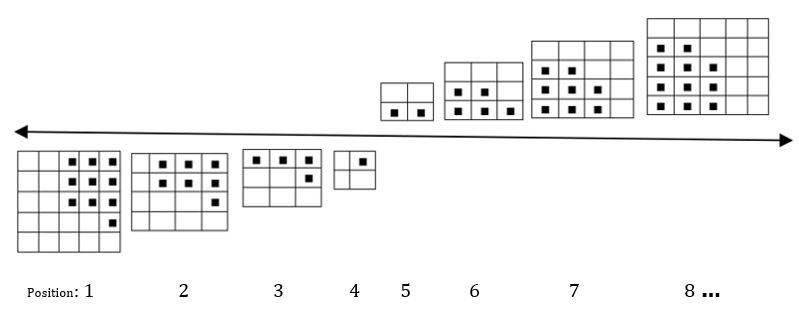1.1 The square grids below the continuous line depict negative numbers and those above the line positive numbers. Study the square grids shown above with black squares forming a pattern. How is the pattern growing? Create square grids 9, 10 and 11; complete them with black squares to further develop the pattern.

1.2 How many small black squares are in the grids in the 9th, 10th and 11th positions?

1.3 Can you predict the number of small black squares in the grids in the 50th and 100th positions?

1.4 What numerical number pattern can be formed from the black squares in each grid?

1.5 Obtain the general term, Tn, for this number pattern.

1.6 What is the mathematical name given to this kind of number pattern?

1.7 If there are 602 black squares in a square grid, determine n, the position of the square grid.